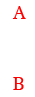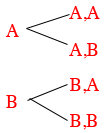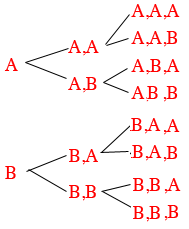SEARCH HOMEMath Central Quandaries & QueriesQuestion from Javar: How many different combinations can I make with A 12 selection list but only using A&B Example: A,B,A,B,A,B,A,B,A,B,A,B A,B,B,A,A,B,B,A,A,B,B,A B,B,A,A,B,A,B,A,B,A,A,BHi Javar,

Combination is a technical word in mathematics so I am going to refer to your examples as strings of letters, each string being 12 letters long and only containing the letters A and B.

Sometimes a productive approach to a problem is to consider a smaller problem and examine it. In this case by smaller problem I am thinking of shorter strings. Lets start with the case of strings that are only 2 letters long and again containing only the letters A and B. There are only 2 of them. A and B. Here they are displayed.What about strings of length 2 letters? Reading from left to right, each string of length 1 can be extended to a string of length 2 by adding either an A or a B. Here are the strings of length 2.Each string of length 2 can be extended to 2 strings of length 2 and hence there are $2 \times 2 = 2^2 = 4$ strings of length 2.

What about strings of length 3 letters? Each of the strings of length 2 can be extended to a string of length 3 by adding either an A or a B so there are $4 \times 2 = 2^2 \times 2 = 2^3 = 8$ strings of length 3. Here they are.How many strings are there of length 12?

PennyMath Central is supported by the University of Regina and The Pacific Institute for the Mathematical Sciences.